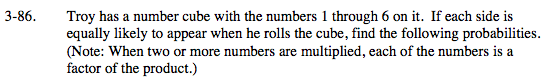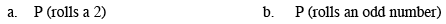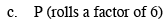### Home > MC2 > Chapter 3 > Lesson 3.2.3 > Problem3-86

3-86.Do you remember how to express probability?

$\frac{\# \text{ of 2's}}{\text{total \#'s possible}}$

How many 2's does the number cube have? How many total numbers does the number cube have?

$\frac{1}{6}$

See (a).

How many odd numbers are on the number cube?List all the factors of 6.

Factors of 6 include: 1, 2, 3, 6

$\frac{4}{6} = \frac{2}{3}$